# On Simulation of the Natural Convection Heat Transfer Between Circular Cylinder and an Elliptical Enclosure Filled with Nanofluid [Part I: The Effect of MHD and Internal Heat Generation/Absorption]

On Simulation of the Natural Convection Heat Transfer Between Circular Cylinder and an Elliptical Enclosure Filled with Nanofluid [Part I: The Effect of MHD and Internal Heat Generation/Absorption]
Air conditioning and Refrigeration Techniques Engineering Department, Al-Mustaqbal University College, Babylon 51001, Iraq

Corresponding Author Email:
Page:
599-610
|
DOI:
https://doi.org/10.18280/mmep.060416
12 July 2019
|
Accepted:
21 November 2019
|
Published:
24 December 2019
| Citation

OPEN ACCESS

Abstract:

I demonstrated numerically the natural convective heat transfer between inner heated circular cylinder located within cooled elliptical enclosure filled with copper-water nanofluid with internal heat generation/absorption in the presence of horizontal magnetic field. The dimensionless governing equations are solved numerically using finite element scheme. The considered parameters of this study Rayleigh number (103<Ra<107), Hartmann number (0<Ha<60), nanofluid volume fraction (0<ϕ<0.06), heat generation/absorption (-10<q<+10) and the horizontal position of the inner circular cylinder (-0.2≤δ≤+0.2). The results show that increasing Rayleigh number and nanofluid volume fraction increases the fluid flow strength and heat transfer rate. While Hartmann number increasing leads to reduce the Nusselt number. It is obtained also, that absorption of heat augments the heat transfer. Finally, it is found that when the circular cylinder moves into the left side, a better heat transfer will be obtained while it is recommended to move the inner cylinder into right for better fluid flow strength

Keywords:

MHD, heat generation/absorption, nanofluid, elliptical enclosure, natural convection

1. Introduction

The natural convection heat transfer heat transfer inside enclosure has wide applications in engineering. These applications include the solar collectors, cooling of building and electronic equipment, micro-electromechanical system, heat exchangers, metallurgical industry and geothermal applications are few examples on natural convective within enclosures. For more detailed regarding these applications the readers can referred to [1-4]. The conventional fluids of heat transfer have serious limitation which it is the low thermal conductivity and this is considered to be a critical challenge for the researchers among the world. One of the ways to enhance the heat transfer is to added nanoparticle which have higher thermal conductivity to the base fluid like water, oil. The resulted fluid is known as nanofluid . Many researchers examined the influence of nanofluid on enhancing the heat transfer rate within different simple shapes of enclosures like square, rectangular, triangular, rhombus, trapezoidal, etc. [6-12]. Abu-Nada and Oztop  examined numerically the natural convection inside inclined square enclosure filled with Cu-O nanofluid. They obtained that the lower heat transfer enhancement at 90o. Morcione  considered that the nanofluid is behaved as a single phase flow rather than solid-liquid mixture mode for natural convection within rectangular enclosure.

The existence of inner body within enclosures effects on the heat transfer rate obviously and many researchers studied the phenomenon of natural convection between inner body located inside enclosure for different shapes and position. Some of these studies like Lee et al.  who studied numerically the influence of the inner isotherm hot circular cylinder located inside isotherm cold square enclosure for various values of Rayleigh numbers with various horizontal and diagonal inner body position. The space between them is filled with air. The major finding is that increasing Rayleigh number augments the heat transfer. Park et al.  examined numerically the influence of vertical position of cold and hot circular cylinder located within square enclosure. Yoon et al.  examined the influence of the radius of two horizontal cylinder located inside square enclosure. They obtained that as the radius reduces, the natural convection effect increases. Kalyana et al.  developed a correlation for Nusselt number in terms of aspect ratio for the natural convection between inner heated elliptical body located inside square enclosure. The results indicated that increasing both Rayleigh number and aspect ratio enhanced the heat transfer rate significantly. Nabavizadeh et al.  examined the natural convection between sinusoidal cylinder inside square enclosure and the results indicated that the Nusselt number might be enhanced or decreased when the number of undulation increases. Bararnia et al.  studied the natural convection between inner elliptical body inside square enclosure. Their findings illustrated that Rayleigh number and the position of inner elliptical body highly effect on the heat transfer and fluid flow characteristics. Abadi et al.  studied the natural convection from two elliptical inner bodies located inside enclosure for different spacing using finite element method. The results showed that the Rayleigh number and the position of inner bodies effect of streamlines and isotherms pattern. Ghasemi et al.  demonstrated the natural convection between circular cavity and inner elliptical body using CVFEM. They found that the size of inner elliptical body is highly effects on the fluid flow circulation. Beside that Rayleigh number is considerably effect on heat transfer rate. Finally, inner elliptical body inclination angle influences on heat transfer rate very much. Another important study presented by Mehrizi et al.  for the natural convection between triangular inner body located within elliptical enclosure for different Rayleigh number, inner body size, aspect ratio and outer elliptical cylinder orientation. The results reveal that the vertical orientation gives higher heat transfer rate more than the horizontal orientation. Farooq et al.  examined the nanofluid mixed convection between inner corrugated cylinder inside outer rotating circular cylinder using finite element method.

The influence of magnetic field for the subject of natural convection within enclosure is studied by various researchers. Ghasemi et al.  examined the magnetic field impact on the buoyancy driven flow in a nanofluid filled square enclosure. They concluded that increasing of Rayleigh number augments the heat transfer rate while increasing of Hartmann number reduces it. The nanofluid volume fraction may increases or decreases the performance depending upon the Rayleigh and Hartmann number. Sheikholeslami et al.  examined the natural convection between circular cylinder inside nanofluid filled square enclosure in the presence of radian magnetic field using lattice-Boltzmann approach. They found that Nusselt number increases as nanofluid volume fraction and Rayleigh number increases while it decreases as the Hartmann number increases. Sheikholeslami et al.  examined the magnetic field effect on the natural convective between inner sinusoidal inner body and outer circular cylinder. They obtained that Nusselt number reduces as Hartmann number increases while it increases as undulation number, nanofluid and Rayleigh number. Another study for Sheikholeslami et al.  examined the magnetic field effect on the natural convection in inclined half-annulus close space (cavity) filled with Cu-O nanofluid. It is obtained that at Ra = 105, the augmentation in the heat transfer at inclination angle 90o is greater than that of 0o and 45o. Sheikholeslami and Rashidi  examined numerically the influence of space dependent magnetic field of natural convection. It is obtained that increasing magnetic number, nanofluid volume fraction and Rayleigh number augments the heat transfer rate but Nusselt number reduces as Hartmann number goes up. Sheikholeslami et al.  examined the nanofluid of Fe3O4 filled elliptical enclosure considering the magnetic field and thermal radiation. It is obtained that Nusselt number enhanced for larger radiation parameter and forces of buoyancy. Tayebi et al.  examined the influence of eccentricity for the natural convection between two annulus enclosure filled with nanofluid flow. The results indicate that the heat transfer can be enhanced by increasing Rayleigh number, but its impact will be more pronounced as the gap between the elliptical cylinders becomes larger. Sheikholeslami et al.  examined the MHD within a nanofluid cubical enclosure with an inner elliptical obstacle filled with porous medium

From the above previous comprehensive review it can be seen that there is a limitations in the previous studies that examined the influence of both MHD and heat generation or/and absorption in an elliptical nanofluid enclosure. So that this paper will be the first try to explain this phenomenon. Finite element scheme had been used for solving the dimensionless governing equations of mass, momentum and energy under the steady state conditions. The considered parameters are Rayleigh number (103<Ra<107), Hartmann number (0<Ha<60), nanofluid volume fraction (0<ϕ<0.06), heat generation/absorption (-10<q<+10) and the horizontal position of the inner circular cylinder ( $-0.2 \leq \delta \leq+0.2$ ).

2. Mathematical Model

2.1 Geometry definition

The schematic representation of the present problem is presented in Figure. 1. The considering system concludes of circular cylinder located within elliptical enclosure. The radius of the inner circular cylinder is R = 0.1. The length of the major axis of the elliptical enclosure is 1 and the minor axis is 0.5. The inner circular cylinder is kept at isotherms hot temperature while the elliptical wall is considered to be kept at cold temperature. The magnetic field is horizontally applied with uniform strength BO. The space between the cylinder and the elliptical enclosure is filled with CuO nanofluid in the presence of heat generation or absorption. The fluid is considered to be incompressible, Newtonian, laminar nature under the steady state conditions. Constant thermphysical properties except the density in the Y-direction of Momentum equation in which Boussinesq approximation had been applied to treat with the density. The thermophysical properties illustrated in Table 1.

## 1.pngFigure 1. The schematic diagram of the inner circular cylinder within the elliptical enclosure

2.2 Governing equations

The mass, momentum and energy laws for non-dimensional laminar natural convection fluid flow with the Boussinesq approximation in y-direction are as following :

$\frac{\partial U}{\partial X}+\frac{\partial V}{\partial Y}=0$   (1)

$U \frac{\partial U}{\partial X}+V \frac{\partial U}{\partial Y}=-\frac{1}{\rho_{\text {naf}}} \frac{\partial P}{\partial X}+\frac{\mu_{\text {naf}}}{\rho_{\text {naf}}}\left(\frac{\partial^{2} U}{\partial X^{2}}+\frac{\partial^{2} U}{\partial \mathrm{Y}^{2}}\right)$   (2)

\begin{aligned} U \frac{\partial V}{\partial X}+V \frac{\partial V}{\partial Y} &=-\frac{\partial P}{\partial Y}+\frac{\mu_{n a f}}{\rho_{n a f} \alpha_{f l}}\left(\frac{\partial^{2} V}{\partial X^{2}}+\frac{\partial^{2} V}{\partial Y^{2}}\right)-\\ & \frac{\rho_{f l} \sigma_{n a f}}{\rho_{n a f} \sigma_{f l}} H a^{2} P r V+\frac{\beta_{n a f}}{\beta_{f l}} R a P r \theta \end{aligned} (3)

$U \frac{\partial \theta}{\partial x}+V \frac{\partial \theta}{\partial Y}=\frac{\alpha_{\operatorname{naf}}}{\alpha_{f l}}\left(\frac{\partial^{2} \theta}{\partial X^{2}}+\frac{\partial^{2} \theta}{\partial Y^{2}}\right)+\frac{\alpha_{\operatorname{naf}}}{\alpha_{f l}} q \theta$ (4)

the dimensionless parameters can be written as below:

$X=\frac{x}{L} ; Y=\frac{y}{L} ; U=\frac{u L}{\alpha_{f l}} ; V=\frac{v L}{\alpha_{f l}}$

$P=\frac{p L^{2}}{\rho_{f l} \alpha_{f l}} ; \theta=\frac{T-T_{c}}{T_{h}-T_{c}}$

$\alpha_{n a f}=\frac{k_{n a f}}{\rho_{n a f} C_{p_{n a f}}} ; \operatorname{Pr}=\frac{v_{f l}}{\alpha_{f l}} ; R a=\frac{g \beta_{f l}\left(T_{h}-T_{c}\right) L^{3}}{\alpha_{f l} v_{f l}}$

$q=\frac{Q_{o} L^{2}}{\left(\rho C_{p}\right)_{n a f}} ; H a=B L \sqrt{\frac{\sigma_{f l}}{\rho_{f l} v_{f l}}}$  (5)

2.3 Thermophysical properties of nanoﬂuid

The thermos-physical properties of the nanofluid can be calculated as follows, respectively [32-37].

$\rho_{n a f}=(1-\emptyset) \rho_{b f l}+\emptyset \rho_{s o l}$ (6)

$\left(\rho C_{p}\right)_{n a f}=(1-\emptyset)\left(\rho C_{p}\right)_{b f l}+\emptyset\left(\rho C_{p}\right)_{s o l}$ (7)

$(\rho \beta)_{n a f}=(1-\emptyset)(\rho \beta)_{b f l}+\emptyset(\rho \beta)_{s o l}$ (8)

The thermal conductivity of the nanofluid according to the Maxwell formula can be written as below:

$k_{\text {naf}}=\frac{\left(k_{\text {sol}}+2 k_{\text {bfl}}\right)-2 \emptyset\left(k_{\text {bfl}}-k_{\text {sol}}\right)}{\left(k_{b f l}+2 k_{\text {sol}}\right)+\emptyset\left(k_{b f l}-k_{s o l}\right)} k_{b f l}$ (9)

The effective dynamic viscosity of the nanofluid the Brinkman model is employed as:

$\mu_{n a f}=\frac{\mu_{b f l}}{(1-\emptyset)^{2.5}}$ (10)

Table 1. Thermo-physical properties of the base fluid as well as the nanoparticles 

 Properties Copper Pure fluid Cp (J/kg k) 385 4179 ρ (kg/m3) 8933 997.1 k (W/m.k) 401 0.613 β(1/k) 1.67×10-5 21×10-5 µ   (kg/m.s) ---- 0.000372

The local and average Nusselt number around cylinder are calculated as below:

$N u_{l o c}=\frac{\partial \theta}{\partial n} ; N u_{a v e}=\frac{1}{2 \pi} \int_{0}^{2 \pi} N u_{l o c}(\varphi) d \varphi$   (11)

2.4 Boundary conditions

The cylinder is located in the center of the elliptical enclosure. The non-dimesional boundary conditions are given below:

The outer ellipse wall is kept at isotherms cold temperature:

$U=U_{n a f}=V=V_{n a f}=\Psi=0 ; \theta=0$

The hot circular cylinder is kept at isotherms hot temperature:

$U=U_{n a f}=V=V_{n a f}=\Psi=0 ; \theta=1$

3. Code Validation

In order to check the accuracy of the present numerical results, it is necessary to validate the present numerical programme with previous published works. So that the validation is presented in terms of streamlines, isotherms s illustrated in Figure 2a. The valuation is done with Kim et al.  who examined numerically the natural convection between inner circular cylinder within square enclosure. Besides that, the validation is presented also with the same researcher in terms of average Nusselt number for low and high value of Rayleigh numbers illustrated in Figure 2b. The validation shows a good agreement with the previous work in terms of contours and Nusselt number. It is worthy to mention that mesh independent study is presented in Table 2 and draw in Figure 3a. It can be seen that Finer mesh gives approximately the same results with the same accuracy for that of extremely fine. Hence, finer mesh is considered for the next calculations. Figure 3b examines the finer mesh for the elliptical enclosure with internal circular cylinder.

## 2a.jpg（a）

## 2b.jpg(b)

Figure 2. a) Validation of Isothermals and streamlines of the present work (bottom) with Kim et al.  (top); b) validation of the average Nusselt number with respect to the Rayleigh number with Kim et al. 

Table 2. Number of element in this study and the average Nusselt number

 Predefined mesh size Number of elements Average Nusselt number Extra coarse 346 2.8996 Coarser 570 3.0699 Coarse 852 3.1272 Normal 1448 3.1478 Fine 2230 3.1509 Finer 3644 3.1466 Extra fine 10178 3.1465 Extremely fine 39306 3.1465

## 3.jpg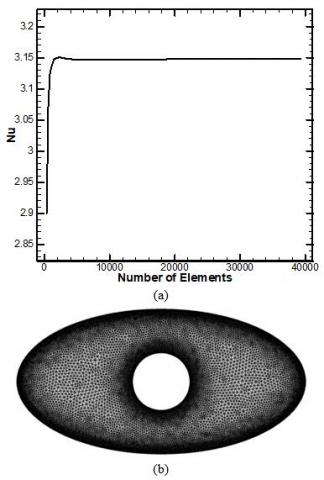Figure 3. a) Mesh independent study in terms of Nusselt number; b) finer mesh of the elliptical enclosure with n internal circular cylinder

4. Results and Discussions

4.1 Influence of Hartman number and Rayleigh number

First of all, a brief discussion for the impact of two-important dimensionless numbers like Hartamnn and Rayleigh number will be presented in terms of streamlines, isotherms, in addition to the local as well as average Nusselt number at Qg = 4, Pr = 6.2 and $\emptyset=0.03$ . it is obvious based on Figure 4a that as the value of Rayleigh number increases, the intensity which it is presented in terms of stream function increases, also due to increasing of the natural convection intensity. This is obvious when the Rayleigh number increases from Ra = 105 into Ra = 107, the stream function increases from Ψ = 3.6 into Ψ = 23. Unlike, as the Hartmann number increases, the intensity of the fluid between the circular heated cylinder and the cooled elliptical enclosure decreases. For example, at Ha = 0, Ψ = 23 while as the Hartmann number goes up into Ha = 60, Ψ = 17. The reason behind this is that increasing the MHD (electromagnetic force) will resist the movements of the fluid particles which reduces its velocity and thus the intensity of the fluid reduces. The impact of those two important parameters is still important on isotherms as illustrated in Figure 4b. The impact of Rayleigh number is very strong as it completely changes the isotherms lines shapes from uniform at low Rayleigh number in which conduction heat transfer is dominated into more horizontal curves at high Rayleigh number value which is indicator of convection mode will be dominated in the transfer of heat between the hot circular cylinder and the cooled elliptical enclosure. The impact of Hartmann number on isotherms is less than that of the Rayleigh number. Secondly, to explain the influence of these important parameters (Ra and Ha), it is necessary to discuss their effect on the Nusselt number which is the indicator dimensionless number for augmentation of heat transfer. Figure 5 shows that the Hartmann number effect is approximately negligible when Rayleigh number is low (less than Ra = 104) while as the Rayleigh number increases, the Hartmann number impact will be more obvious. It can be seen that Harmann number increasing leads to decreases in the average Nusselt number which reduces the heat transfer rate. On the other hand, Rayleigh number increasing enhances the Nusselt number. For more concentration on the Hartmann number effect, an explanation for the impact of Hartmann number and Rayleigh number on the local Nusselt number along the inner circular cylinder is presented in Figure 6 at high value of Rayleigh number. It proved what explained before in Figure 5 which the Rayleigh and Hartmann number have adverse impact on the Nusselt number.

## 41.png## 42a.png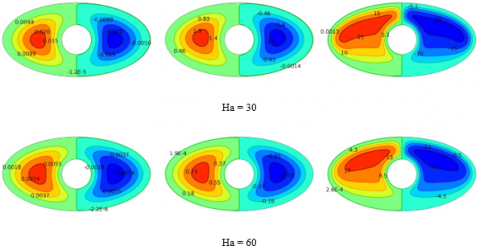(a)

## 4b.png(b)

Figure 4. a) Streamlines contours for various values of Rayleigh and Hartmann numbers; b) isotherms contours for various values of Rayleigh and Hartmann numbers

## 5.pngFigure 5. Impact of Hartmann and Rayleigh number on average Nusselt number

## 6.png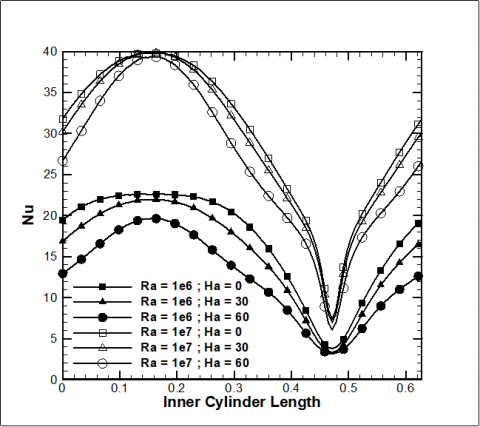Figure 6. Local Nusselt number profile along the circular cylinder under various Hartmann and Rayleigh number

4.2 Influence of heat generation/absorption and Rayleigh number

As the present work focus on the influence of generation/absorption in addition to the magnetic field, a full-details explanation will be presented in terms of contours of streamlines as well as the isotherms, local and average Nusselt number. Figure 7a explains the streamlines under various Rayleigh number and heat coefficient for two cases (generation/absorption). Firstly, at low Rayleigh number (Ra = 103). For case 1 which is the heat absorption where q<0; as the heat coefficient goes up from q=-5 into =-10, the stream function goes down from Ψ = 0.019200 into Ψ = 0.018919, respectively. On the other hand, for the case number 2 which is the heat generation conditions in which q>0; when the heat coefficient increases from q=5 into q=10, the stream function increases from Ψ = 0.01838 into Ψ = 0.020179. It may be noted that the influence of heat coefficient is still small in low Rayleigh number. Secondly, at High Rayleigh number for example at Ra = 107, the stream function decreases from Ψ =20.503 at =-5 into Ψ = 20.490 at q=-10 (heat absorption case). While for the case of heat generation, the stream function increases from Ψ = 20.572 at q=5 into Ψ = 20.620 at q=10. Thus, the impact of heat coefficient is approximately negligible at high Rayleigh number. The influence of heat coefficient on the isotherms is presented on Figure 7b and it can be seen that impact of Rayleigh number is higher than the impact of heat coefficient. As the Nusselt number is important on heat transfer rate, Figure 8 explains the impact of heat coefficient on average Nusselt number with respect to the Rayleigh number. Obviously at low Rayleigh number, when the heat coefficient goes from the negative value (absorption case) into the positive vale (generation case), average Nusselt number decreases. So that it is recommended that absorption case play an important role in enhancing the heat transfer. However, at high Rayleigh number the heat coefficient is negligible. Now, the influence of heat coefficient on local Nusselt number profile along the inner circular cylinder at Ra = 105 is displayed on Figure 9. It can be seen the profile of local Nusselt number at q=-10 is higher than that at q=10. As the present work examines the impact of magnetic field and heat generation / absorption, an explanation is presented in Figure 10 at Ra = 105. As discussed before, increasing Hartmann number, reduces the average Nusselt number. Also, the Nusselt number increases as the heat coefficient decreases (heat absorption case).

## 7a1.png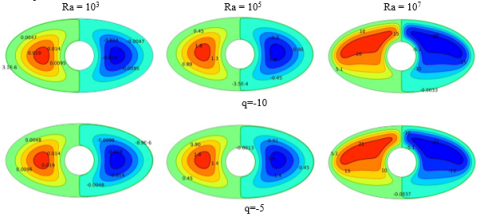## 7a2.png(a)

## 7b1.png## 7b2.png(b)

Figure 7. a) Streamlines contours for various values of Rayleigh and heat coefficient; b) isotherms contours for various values of Rayleigh and heat coefficient

## 8.pngFigure 8. Nusselt number variation with Rayleigh number under different values of heat coefficient

## 9.pngFigure 9. Local Nuselt number profile along the circular cylinder for various heat coefficient

## 10.png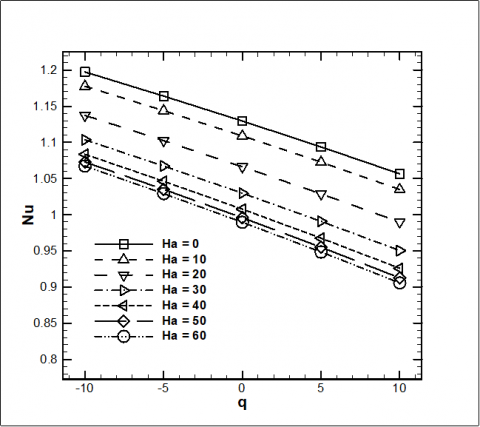Figure 10. Impact of Hartmann number and heat coefficient on the average Nusselt number

4.3 Influence of nanofluid volume fraction

Nanofluid play an important role in augmentation of heat transfer so that this section of this study will be focused on it. Figure 11a and 11b demonstrates the impact of the nanofluid loading ( $\emptyset$= 0, 0.02, 0.04 and 0.06) on the average Nusselt number for various heat coefficient and Hartmann number. It may be noted that the Nusselt number increases as the loading of the nanofluid increases which leads to enhance the heat transfer.

## 11a.png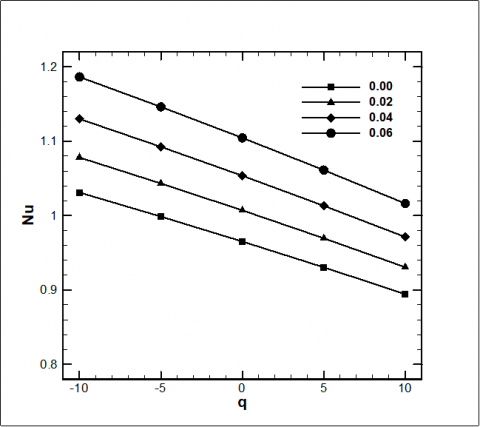(a)

## 11b.png(b)

Figure 11. a) Average Nusselt number for various nanofluid loading and heat coefficient; b) Average Nusselt number for various nanofluid loading and Hartmann number

4.4 Influence of horizontal position of inner circular cylinder

This section examines the influence of the inner circular cylinder horizontal position on the fluid flow and heat transfer. Two cases are considered which they are when the inner body move horizontally to the left side of the side of the elliptical enclosure and when it moves into the right side at low and high Rayleigh numbers. Firstly, the discussion will be in terms of streamlines and isotherms as illustrated in Figure 12. It can be seen that when the inner body moves into the left side, it compress the nanofluid into the left side and reduces the fluid intensity in that direction (i.e., in the direction when the inner body moves to it) which increases the fluid intensity in the other side (right side). In the present section, we consider the stream function on the left side to examine the impact of the horizontal movements on the flow intensity. For example, at low Rayleigh number when the cylinder moves from the center of the enclosure into the left side, the stream function decreases from Ψ=0.019838 into Ψ=0.0046509. It can be seen that when the inner body is located in the center of the elliptical enclosure, the streamlines contours has stable shape and the magnitude of the stream function is the same in both sides.  Now, when the cylinder moves into the right direction, the stream function increases into Ψ=0.032303.

## 12.pngFigure 12. Streamlines and isotherms for various inner circular position at Ra = 103 and Ra = 107

This behavior is the same at high Rayleigh number but with larger magnitude of stream function. The magnitude and the horizontal location impacts strongly on the isotherms contours as illustrated in Figure 12 where the position completely changes the hottest zones between the inner circular body and the enclosure. The hottest zones will be in the region where the cylinder move to it. However, the isotherms contours is stable when the cylinder located in the center of the enclosure.

The impact of the position on Nusselt number is presented in Figure 13a and Figure 13b. it can be seen that when the Rayleigh number is less that 105 and the cylinder moves into the left side there is no effect or small effect on Nusselt number. While there is a clear change in the magnitude of the Nusselt number as the cylinder moves into the right direction. While at very high value of Rayleigh number, when the cylinder moves into the left side, it will have the largest value of Nusselt number which leads to more heat transfer enhancement.

## 13a.png(a)

## 13b.png(b)

Figure 13. a) Average Nusselt number for Rayleigh number less than 105 at different inner circular position; b) Average Nusselt number for high Rayleigh number at different inner circular position

5. Conclusions

The present work examines numerically the natural convection between inner heated circular cylinder located within nanofluid elliptical enclosure using finite element scheme. The main conclusions indicate that increasing of Rayleigh number and nanofluid loading leads to enhance both the intensity of the fluid flow and heat transfer. While, Increasing Hartmann number reduces the fluid intensity and the heat transfer as it resists the fluid motion. Also, Heat absorption conditions gives better heat transfer characteristics more that the generation conditions. It is recommended to moves the inner circular cylinder horizontally to the left side for better heat transfer and moves it into the right side for better fluid flow strength. Finally, it is obtained that at high value of Rayleigh number (Ra = 107), the Nusselt number decreases as the inner cylinder moves from the left to the right.

Acknowledgment

I would like to thanks the Editorial Team of the Journal of Mathematical Modelling of Engineering Problems for their efforts and the respected reviewer for his comments that clearly enhance the quality of the manuscript.

Nomenclature
 B Magnetic field Cp Specific heat at constant pressure (kJ/kg.K) g Gravitational acceleration (m/s2) k Thermal conductivity (W/m.K) P Dimensionless pressure Pr Prandtl number (νf/αf) Ro Base circle (m) Ra Rayleigh number $\mathrm{g} \beta_{\mathrm{f}} \mathrm{L}^{3} \Delta \mathrm{T} / v_{\mathrm{f}} \alpha_{\mathrm{f}}$ T Temperature (K) Tc Temperature of the cold surface (K) Th Temperature of the hot surface (K) q Heat coefficient Nu Average Nusselt number hot inner circular cylinder U Dimensionless velocity component in x-direction u Velocity component in x-direction (m/s) V Dimensionless velocity component in y-direction v Velocity component in y-direction (m/s) X Dimensionless coordinate in horizontal direction x Cartesian coordinates in horizontal direction (m) Y Dimensionless coordinate in vertical direction y Cartesian coordinate in vertical direction (m) Greek symbols α Thermal diffusivity (m2/s) θ Dimensionless temperature (T-Tc/ΔT) $\mathbf{\psi}$ Dimensional stream function (m2/s) Φ Angle of circular cylinder $\mathbf{\psi}$ Dimensionless stream function Gr Grashof number μ Dynamic viscosity (kg/m.s) ν Kinematic viscosity (m2s-1) β Volumetric coefficient of thermal expansion (K-1) ρ Density (kg/m3) Subscript c Cold f Fluid (pure) h hot
References

 Bejan, A. (2013). Convection Heat Transfer. John Wiley & Sons. http://dx.doi.org/10.1002/9781118671627

 Ostrach, S. (1988). Natural convection in enclosures. Journal of Heat Transfer, 110(4b): 1175-1190. http://dx.doi.org/10.1115/1.3250619

 Majdi, H.S., Abdulkadhim, A., Abed, A.M. (2019). Numerical investigation of natural convection heat transfer in a parallelogramic enclosure having an inner circular cylinder using liquid nanofluid. Frontiers in Heat and Mass Transfer (FHMT), 1-14. http://dx.doi.org/10.5098/hmt.12.2

 Abdulkadhim, A., Abed, A.M., Mohsen, A.M., Al-Farhany, K. (2018). Effect of partially thermally active wall on natural convection in porous enclosure. Mathematical Modelling of Engineering Problems, 5(4): 395-406. https://doi.org/10.18280/mmep.050417

 Choi, S.U., Eastman, J.A. (1995). Enhancing thermal conductivity of fluids with nanoparticles. 1995 International Mechanical Engineering Congress and Exhibition, San Francisco, CA, United States.

 Abu-Nada, E., Oztop, H.F. (2009). Effects of inclination angle on natural convection in enclosures filled with Cu–water nanofluid. International Journal of Heat and Fluid Flow, 30(4): 669-678. https://doi.org/10.1016/j.ijheatfluidflow.2009.02.001

 Corcione, M. (2010). Heat transfer features of buoyancy-driven nanofluids inside rectangular enclosures differentially heated at the sidewalls. International Journal of Thermal Sciences, 49(9): 1536-1546. https://doi.org/10.1016/j.ijthermalsci.2010.05.005

 Saleh, H., Roslan, R., Hashim, I. (2011). Natural convection heat transfer in a nanofluid-filled trapezoidal enclosure. International Journal of Heat and Mass Transfer, 54(1-3): 194-201. https://doi.org/10.1016/j.ijheatmasstransfer.2010.09.053

 Mahmoodi, M., Hashemi, S.M. (2012). Numerical study of natural convection of a nanofluid in C-shaped enclosures. International Journal of Thermal Sciences, 55: 76-89. https://doi.org/10.1016/j.ijthermalsci.2012.01.002

 Rahman, M., Öztop, H.F., Mekhilef, S., Saidur, R., Al-Saleme, K. (2014). Unsteady natural convection in Al2O3–water nanoliquid filled in isosceles triangular enclosure with sinusoidal thermal boundary condition on bottom wall. Superlattices and Microstructures, 67: 181-196. https://doi.org/10.1016/j.spmi.2014.01.001

 Choi, C., Jeong, S., Ha, M.Y., Yoon, H.S. (2014). Effect of a circular cylinder’s location on natural convection in a rhombus enclosure. International Journal of Heat and Mass Transfer, 77: 60-73. https://doi.org/10.1016/j.ijheatmasstransfer.2014.04.071

 Ngo, I.L., Byon, C. (2015). Effects of heater location and heater size on the natural convection heat transfer in a square cavity using finite element method. Journal of Mechanical Science and Technology, 29(7): 2995-3003. https://doi.org/10.1007/s12206-015-0630-z

 Lee, J., Ha, M., Yoon, H. (2010). Natural convection in a square enclosure with a circular cylinder at different horizontal and diagonal locations. International Journal of Heat and Mass Transfer, 53(25-26): 5905-5919. https://doi.org/10.1016/j.ijheatmasstransfer.2010.07.043

 Park, Y.G., Yoon, H.S., Ha, M.Y. (2012). Natural convection in square enclosure with hot and cold cylinders at different vertical locations. International Journal of Heat and Mass Transfer, 55(25-26): 7911-7925. https://doi.org/10.1016/j.ijheatmasstransfer.2012.08.012

 Yoon, H.S., Jung, J.H., Park, Y.G. (2012). Natural convection in a square enclosure with two horizontal cylinders. Numerical Heat Transfer, Part A: Applications, 62(9): 701-721. https://doi.org/10.1080/10407782.2012.709438

 Kalyana Raman, S., Arul Prakash, K., Vengadesan, S. (2012). Natural convection from a heated elliptic cylinder with a different axis ratio in a square enclosure. Numerical Heat Transfer, Part A: Applications, 62(8): 639-658. https://doi.org/10.1080/10407782.2012.707058

 Nabavizadeh, S.A., Talebi, S., Sefid, M., Nourmohammadzadeh, M. (2012). Natural convection in a square cavity containing a sinusoidal cylinder. International Journal of Thermal Sciences, 51: 112-120. https://doi.org/10.1016/j.ijthermalsci.2011.08.021

 Bararnia, H., Soleimani, S., Ganji, D. (2011). Lattice Boltzmann simulation of natural convection around a horizontal elliptic cylinder inside a square enclosure. International Communications in Heat and Mass Transfer, 38(10): 1436-1442. https://doi.org/10.1016/j.icheatmasstransfer.2011.07.012

 Abadi, S.M.A.N.R., Jafari, A. (2012). Investigating the natural convection heat transfer from two elliptic cylinders in a closed cavity at different cylinder spacings. Heat Transfer Research, 43(3): 259-284. https://doi.org/10.1615/HeatTransRes.2012002036

 Ghasemi, E., Soleimani, S., Bararnia, H. (2012). Natural convection between a circular enclosure and an elliptic cylinder using control volume based finite element method. International Communications in Heat and Mass Transfer, 39(8): 1035-1044. https://doi.org/10.1016/j.icheatmasstransfer.2012.06.016

 Mehrizi, A.A., Sedighi, K., Farhadi, M., Sheikholeslami, M. (2013). Lattice Boltzmann simulation of natural convection heat transfer in an elliptical-triangular annulus. International Communications in Heat and Mass Transfer, 48: 164-177. https://doi.org/10.1016/j.icheatmasstransfer.2013.08.009

 Ali, F.H., Hamzah, H.K., Abdulkadhim, A. (2019). Numerical study of mixed convection nanofluid in an annulus enclosure between outer rotating cylinder and inner corrugation cylinder. Heat Transfer—Asian Research, 47(7). https://doi.org/10.1002/htj.21387

 Ghasemi, B., Aminossadati, S., Raisi, A. (2011). Magnetic field effect on natural convection in a nanofluid-filled square enclosure. International Journal of Thermal Sciences, 50(9): 1748-1756. https://doi.org/10.1016/j.ijthermalsci.2011.04.010

 Sheikholeslami, M., Gorji-Bandpay, M., Ganji, D. (2012). Magnetic field effects on natural convection around a horizontal circular cylinder inside a square enclosure filled with nanofluid. International Communications in Heat and Mass Transfer, 39(7): 978-986. https://doi.org/10.1016/j.icheatmasstransfer.2012.05.020

 Sheikholeslami, M., Gorji-Bandpy, M., Ganji, D.D., Soleimani, S., Seyyedi, S.M. (2012). Natural convection of nanofluids in an enclosure between a circular and a sinusoidal cylinder in the presence of magnetic field. International Communications in Heat and Mass Transfer, 39(9): 1435-1443. https://doi.org/10.1016/j.icheatmasstransfer.2012.07.026

 Sheikholeslami, M., Gorji-Bandpy, M., Ganji, D.D., Soleimani, S., Seyyedi, S.M. (2013). Effect of a magnetic field on natural convection in an inclined half-annulus enclosure filled with Cu–water nanofluid using CVFEM. Advanced Powder Technology, 24(6): 980-991. https://doi.org/10.1016/j.apt.2013.01.012

 Sheikholeslami, M., Rashidi, M.M. (2015). Effect of space dependent magnetic field on free convection of Fe3O4–water nanofluid. Journal of the Taiwan Institute of Chemical Engineers, 56: 6-15. https://doi.org/10.1016/j.jtice.2015.03.035

 Sheikholeslami, M., Hayat, T., Alsaedi, A. (2017). On simulation of nanofluid radiation and natural convection in an enclosure with elliptical cylinders. International Journal of Heat and Mass Transfer, 115: 981-991. https://doi.org/10.1016/j.ijheatmasstransfer.2017.07.119

 Tayebi, T., Chamkha, A.J., Djezzar, M., Bouzerzour, A. (2017). Natural convective nanofluid flow in an annular space between confocal elliptic cylinders. Journal of Thermal Science and Engineering Applications, 9(1): 011010. https://doi.org/10.1115/1.4034599

 Sheikholeslami, M., Shah, Z., Shafee, A., Khan, I., Tlili, I. (2019). Uniform magnetic force impact on water based nanofluid thermal behavior in a porous enclosure with ellipse shaped obstacle. Scientific Reports, 9(1): 1196. https://doi.org/10.1038/s41598-018-37964-y

 Teamah, M.A., El-Maghlany, W.M. (2012). Augmentation of natural convective heat transfer in square cavity by utilizing nanofluids in the presence of magnetic field and uniform heat generation/absorption. International Journal of Thermal Sciences, 58: 130-142. https://doi.org/10.1016/j.ijthermalsci.2012.02.029

 Khanafer, K., Vafai, K., Lightstone, M. (2003). Buoyancy-driven heat transfer enhancement in a two-dimensional enclosure utilizing nanofluids. International Journal of Heat and Mass Transfer, 46(19): 3639-3653. https://doi.org/10.1016/S0017-9310(03)00156-X

 Jou, R.Y., Tzeng, S.C. (2006). Numerical research of nature convective heat transfer enhancement filled with nanofluids in rectangular enclosures. International Communications in Heat and Mass Transfer, 33(6): 727-736. https://doi.org/10.1016/j.icheatmasstransfer.2006.02.016

 Oztop, H.F., Abu-Nada, E. (2008). Numerical study of natural convection in partially heated rectangular enclosures filled with nanofluids. International Journal of Heat and Fluid Flow, 29(5): 1326-1336. https://doi.org/10.1016/j.ijheatfluidflow.2008.04.009

 Aminossadati, S., Ghasemi, B. (2009). Natural convection cooling of a localised heat source at the bottom of a nanofluid-filled enclosure. European Journal of Mechanics-B/Fluids, 28(5): 630-640. https://doi.org/10.1016/j.euromechflu.2009.05.006

 Ghasemi, B., Aminossadati, S. (2010). Periodic natural convection in a nanofluid-filled enclosure with oscillating heat flux. International Journal of Thermal Sciences, 49(1): 1-9. https://doi.org/10.1016/j.ijthermalsci.2009.07.020

 Das, D., Roy, M., Basak, T. (2017). Studies on natural convection within enclosures of various (non-square) shapes–A review. International Journal of Heat and Mass Transfer, 106: 356-406. https://doi.org/10.1016/j.ijheatmasstransfer.2016.08.034

 Kim, B., Lee, D.S., Ha, M.Y., Yoon, H.S. (2008). A numerical study of natural convection in a square enclosure with a circular cylinder at different vertical locations. International Journal of Heat and Mass Transfer, 51(7-8): 1888-1906. https://doi.org/10.1016/j.ijheatmasstransfer.2007.06.033